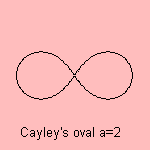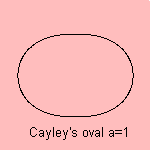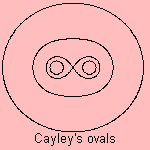# Cayley's oval

## octic

last updated: 2004-02-21The equation is the bipolar equation of the Cayley's oval. Arthur Cayley (1821-1895) studied the curve in 1857.

The curve is formed in nature as the equipotential lines 1) in an electromagnetic or gravitational field.
It is an octic curve 2).And together in one field:notes

1) While the force is of the form F = k/r2, the potential has the form V=k/r.

2) Realize that r12 = (x+1)2 + y2, r22 = (x-1)2 + y2